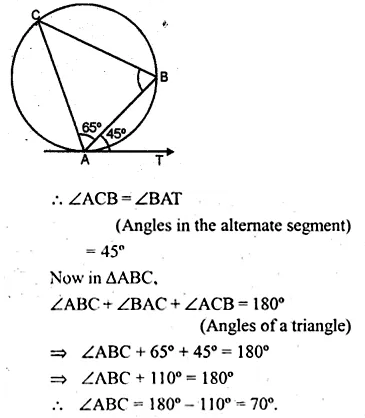Guru

# Question 35. (a) In the figure (i) given below, AT is tangent to a circle at A. If ∠BAT = 45° and ∠BAC = 65°, find ∠ABC.

• 1

One of the most important and exam oriented question from Chapter name- circles
Topic – Angle properties of circles
Chapter number- 15

In this question we have a figure and it is given that AT is tangent to a circle at A.

Also ∠BAT = 45° and ∠BAC = 65°,

Now we have to find ∠ABC.
ICSE Avichal publication
Understanding ICSE Mathematics
Question 35(a)

Share